Latest Banking jobs   »

# Quantitative Aptitude Quiz For Bank Foundation 2023 10th January

Q1. The difference between 40% of y and 20% of x is 270 whereas difference between 40% of x and 20% of y is zero. Find the sum of ‘x’ and ‘y’?
(a) 1250
(b)1400
(c) 1200
(d) 1350
(e)1500

Q2. A retailer buys article A and markup it 20 % above its cost price. At the time of sale if he gave 10% discount instead of 20% and he earns Rs. 4.8 more. Find the cost price of the article A.
(a) 100
(b) 80
(c) 60
(d) 40
(e) 50

Q3. a, b, c and d are four consecutives even numbers, if the sum of ‘a’ and ‘c’ is 120, what is the product of ‘b’ and ‘d’?
(a) 4030
(b) 3780
(c) 3900
(d) 3800
(e) 3840

Q4. Three numbers are given. The average of first and third numbers is 24 more than that of average of second and third numbers. Find out the difference between the first and second numbers.
(a) 36
(b) 40
(c) 42
(d) 48
(e) 46

Q5. If the price of milk is increased by 25% then a person can buy 8 litres less milk by spending Rs 160. Find the final rate of milk?
(a) Rs 4 per litre
(b) Rs 5 per litre
(c) Rs 8 per litre
(d) Rs 6 per litre
(e) Rs 7 per litre

Q6. In an election between P and Q , if 2/5 th of the total voters promised to vote for P and rest promised to vote for Q. On the voting day 25% of the voters went back on their promise to vote for P & 30% of the voters went back on their promise to vote for Q. Find the total no. of voters, if Q wins by 400 votes.
(a) 8000
(b) 10000
(c) 15000
(d) 5000
(e) 12000

Q7. Rahul bought an earphone & paid 10% less than its original price. If he sold it at 20% profit on the price he had bought, what profit percentage did Rahul earn on the original price?
(a) 10%
(b) 6%
(c) 5%
(d) 8%
(e) 12%

Q8. The product of the age of Bhagat and Rahu is 240. If twice the age of Rahu is more than Bhagat’s age by 4 years. Then what is the age of Rahu?
(a) 12 years
(b) 10 years
(c) 15 years
(d) 8 years
(e) 20 years

Q9. A bottle contains three-fourths of milk and the rest water. How much of the mixture must be taken away and replaced by an equal quantity of water so that the mixture has half milk and half water?
(a) 25%
(b) 33.33%
(c) 45%
(d) 50%
(e) 66.67%

Q10. A and B started a business with some amount after 9 months B left the business & C joins the business with Rs. 12,000. And remains in business till the end of year. After one year A, B and C got Rs. 48, Rs. 48 and Rs. 24 respectively. Find the sum of the amount A and B started the business?
(a) 8,000
(b) 10,000
(c) 15,000
(d) 12,000
(e) 14,000

Q11. A trader mixes 26 kg of rice at Rs. 20 per kg. and ‘x’ kg of Rice at Rs. 30 per kg. He sold the mixture at Rs. 39 per kg and earn 50% profit. Find the value of ‘x’?
(a) 30 kg
(b) 39 kg
(c) 32 kg
(d) 36 kg
(e) 42 kg

Q12. The sum of digits of a two-digit number is 12 and the difference between the two-digits of the two-digit number is 6. What is the two-digit number?
(a) 39
(b) 28
(c) 93
(d) 75
(e) Either (a) or (c)

Q13. A man sold article A in 100% profit and article B in 20% profit. If selling price of article, A and article B in same then find his overall profit percentage.
(a) 40%
(b) 50%
(c) 55%
(d) 60%
(e) 75%

Q14. Present average age of a couple is 29 years after 2 year and 4 years respectively a boy child and a girl child are born. Find the average age of family after 8 years of present time?
(a) 18
(b) 19
(c) 20
(d) 21
(e) 22

Q15. The present age of Bhagat and Abhi are in ratio of 9 : 8 respectively. After 10 years the ratio of their ages will be 10 : 9. What is the difference in their present age.
(a) 8 years
(b) 6 years
(c) 12 years
(d) 4 years
(e) 10 years

Solutions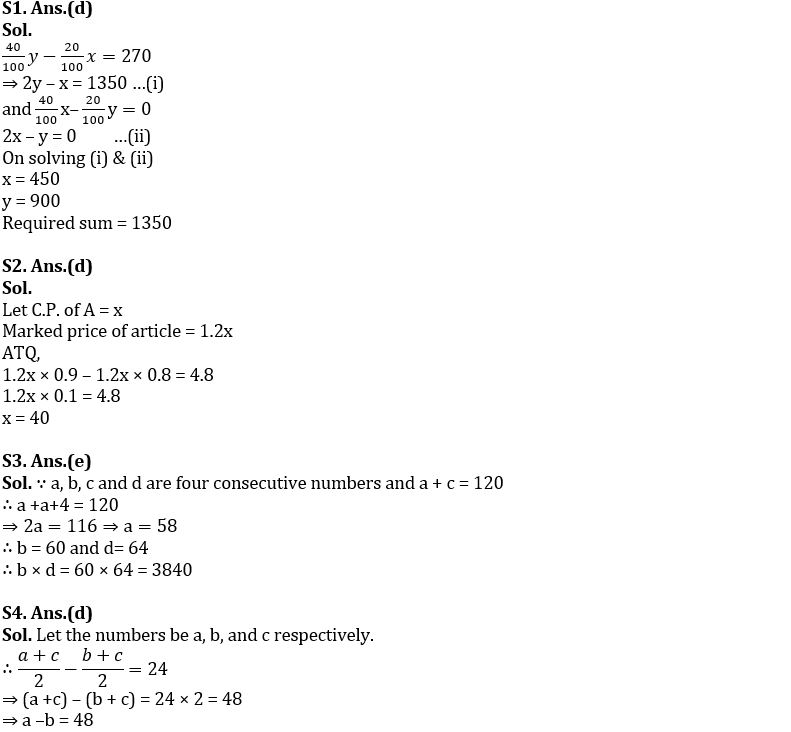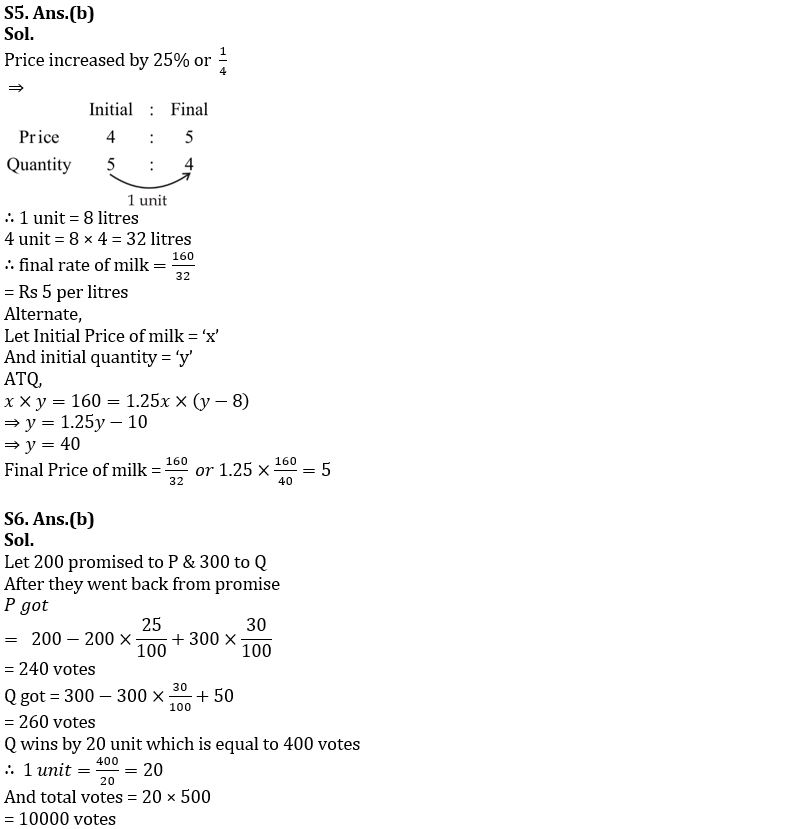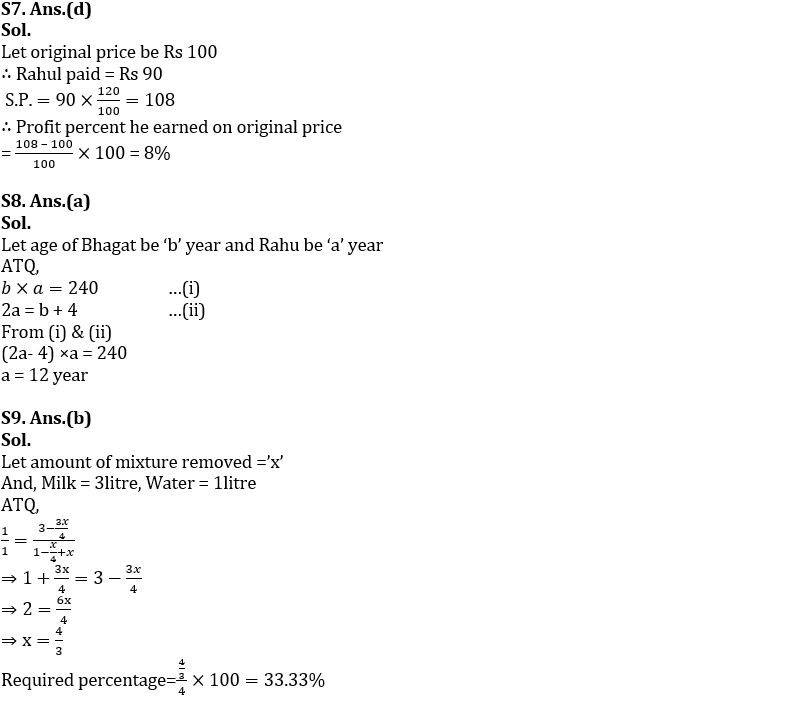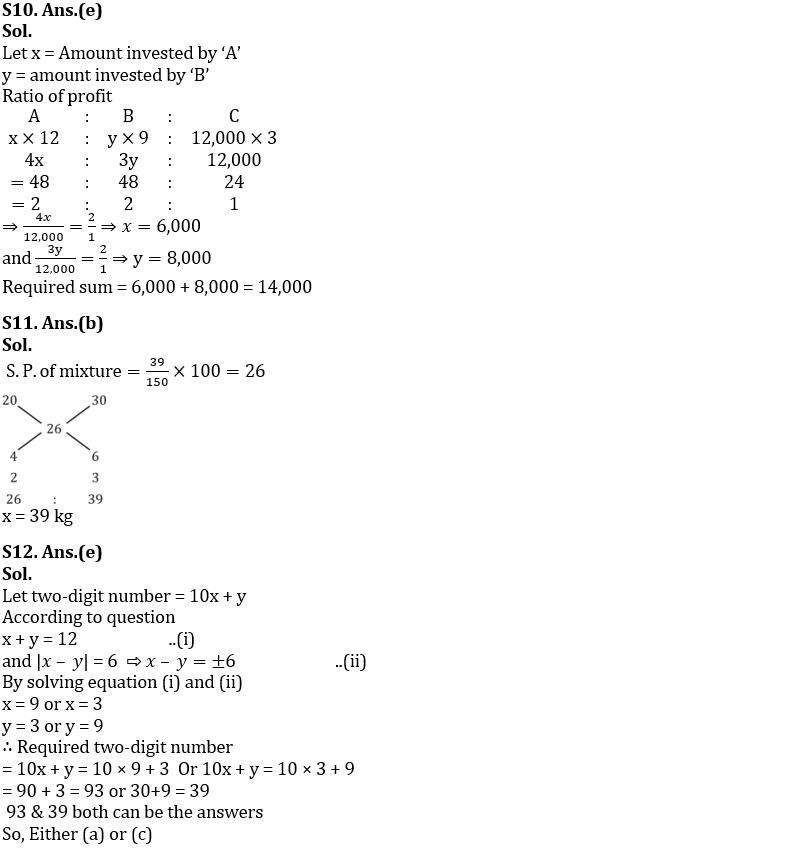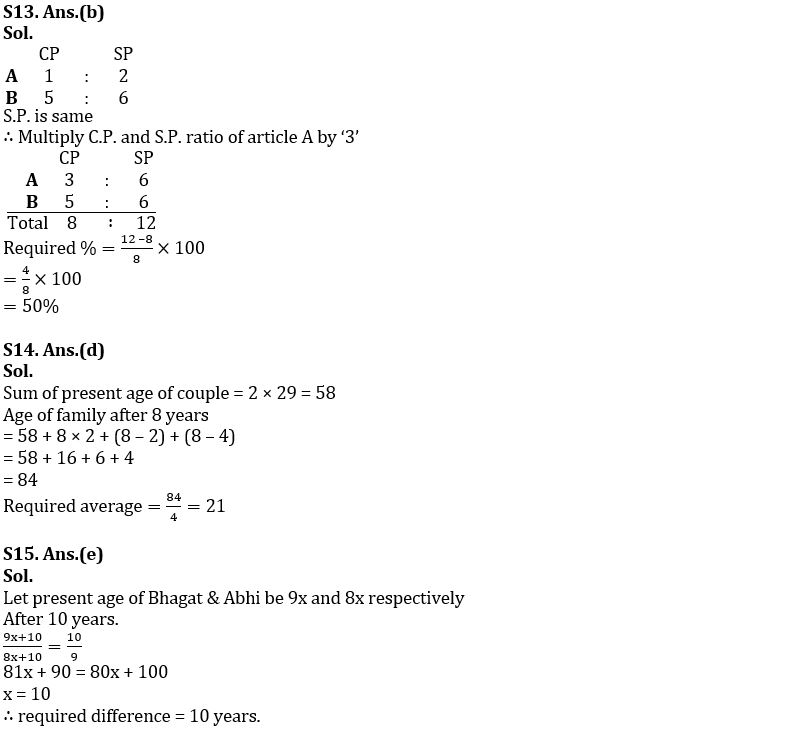## FAQs

### What is the selection process of the Bank Clerk?

The selection process of the Bank Clerk is Prelims & Mains.

#### Congratulations!